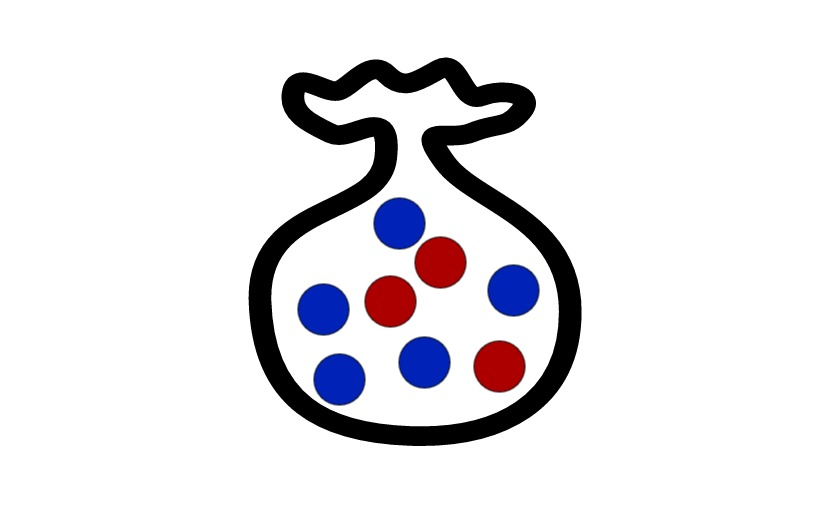# 2.5 Events Without Equally Likely Results

Last updated: February 24th, 2020

# Events Without Equally Likely Results¶

We have said that if the experiment has equally likely results, then $P(E)=\dfrac{|E|}{|\Omega|}$. But, what happens if not?

To see that the formula doesn't hold, let's look at a simple counterexample, and let's see how to sort it out.

Imagine we have a bag with $8$ marbles, $3$ red and $5$ blue. The experiment is taking a marble from the bag and writing its colour.We have $\Omega=\{red,blue\}$, and we can call $R=\{red\}$ and $B=\{blue\}$.

If we think about it with the previous formula, we would have $P(R)=\dfrac{|R|}{|\Omega|}=\dfrac{1}{2}$, and $P(B)=\dfrac{|B|}{|\Omega|}=\dfrac{1}{2}$ which is clearly not the case since there are more blue marbles than red marbles.

Instead, we are going to think about this probability as a proportion: the number of successful cases over the total amount of cases.

Using this idea, we get $P(R)=\dfrac{3}{8}$ and $P(B)=\dfrac{5}{8}$.